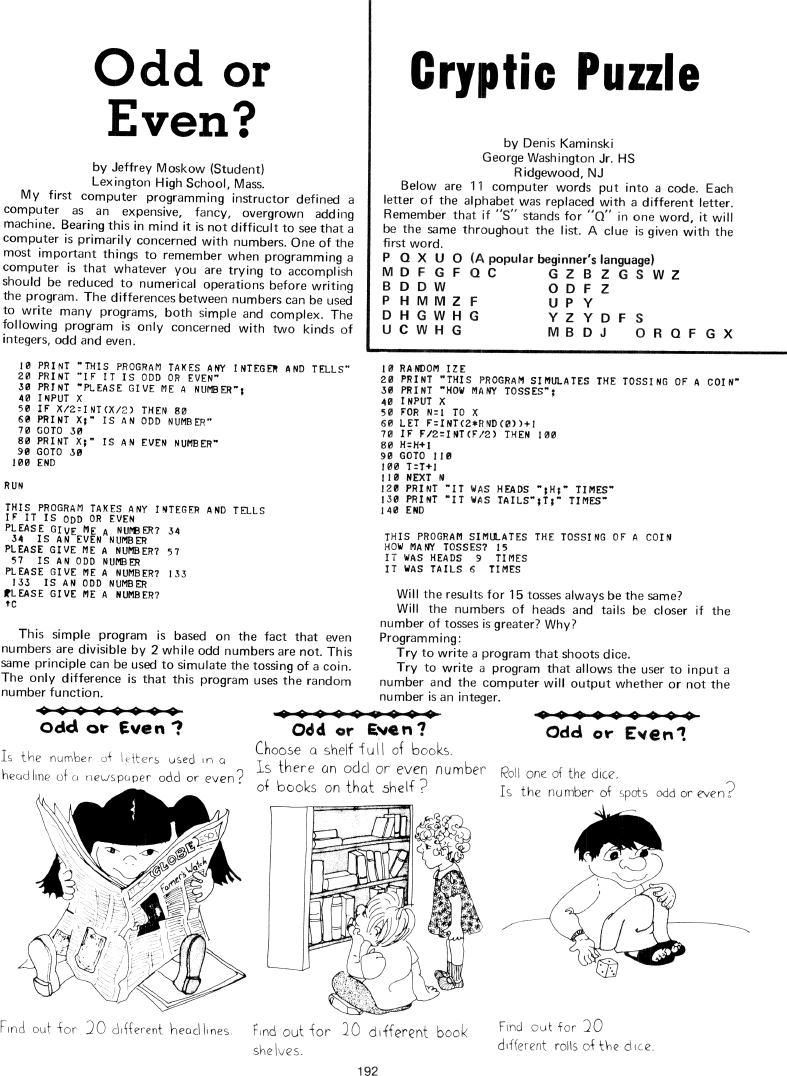The Best of Creative Computing Volume 1 (published 1976)

 `Page 192 << PREVIOUS >> NEXT Jump to page: Go to contents Go to thumbnails`

Odd or Even? and Cryptic Puzzle (Computer puzzles)```Odd or
Even?

by Jeffrey Moskow (Student)
Lexington High School, Mass.

My first computer programming instructor defined a
computer as an expensive, fancy, overgrown adding
machine. Bearing this in mind it is not difficult to see that a
computer is primarily concerned with numbers. One of the
most important things to remember when programming a
computer is that whatever you are trying to accomplish
should be reduced to numerical operations before writing
the program. The differences between numbers can be used
to write many programs, both simple and complex. The
following program is only concerned with two kinds of
integers, odd and even.

10 PRINT "THIS PROGRAM TAKES ANY INTEGER AND TELLS"

20 PRINT "IF IT IS ODD OR EVEN"

30 PRINT "PLEASE GIVE ME A NUMBER";
40 INPUT X
55 IF X/2=INT(X/2) THEN 80
69 PRINT X;" IS AN ODD NUMBER"

75 GOTO 30
83 PRINT X;" IS AN EVEN NUMBER"

99 GOTO 30
100 END

RUN

THIS PROGRAM TAKES ANY INTEGER AND TELLS
IF IT IS ODD OR EVEN
PLEASE GIVE ME A NUMBER? 34
34 IS AN EVEN NUMBER
PLEASE Give ME A NUMBER? 57
57 IS AN ODD NUMBER
PLEASE GIVE ME A NUMBER? 133
133 IS AN ODD NUMBER
+C
This simple program is based on the fact that even
numbers are divisible by 2 while odd numbers are not. This
same principle can be used to simulate the tossing of a coin.

The only difference is that this program uses the random
number function.

Crypiic Puzzle
by Denis Kaminski
George Washington Jr. HS
Ridgewood, NJ
Below are 11 computer words put into a code. Each
letter of the alphabet was replaced with a different letter.

Remember that if "S" stands for "Q" in one word, it will
be the same throughout the list. A clue is given with the
first word.

P Q X U 0 (A popular beginner's language)
M D F G F Q C       G Z B Z G S W Z
B D D W             O D F Z
P H M M Z F         U P Y
D H G W H G         Y Z Y D F S
U C W H G           M B D J   O R Q F G X
10 RANDOM IZE
20 PRINT "THIS PROGRAM SIMULATES THE TOSSING OF A COIN"

30 PRINT "HOW MANY TOSSES" ;
40 INPUT X
50 FOR N=l T0 X
60 LET F=INT(2tRND(D))+l
70 IF' F/2=INT(F/2) THEN 100
80 H=H+l
90 GOTO 110
100 T=T+l
110 NEXT N
l20 PRINT 'IT WAS HEADS ";H;" TIMES"

130 PRINT 'IT WAS TAILS";T;" TIMES"

140 END

THIS PROGRAM STIMULATES THE TOSSING OF A COIN
NON MANY TOSSES? I5
IT WAS TAILS 6 TIMES

Will the results for 15 tosses always be the same?

Will the numbers of heads and tails be closer if the
number of tosses is greater? Why?

Programming:

Try to write a program that shoots dice.

Try to write a program that allows the user to input a
number and the computer will output whether or not the
number is an integer.

Odd or Even?                            Odd or Even?                       Odd
or Even?
Is the number of letters used in a      Choose a shelf full of books.      Roll
one of the dice
headline of a newspaper odd or even?    ls there an odd or even number     Is
the number of spots odd or even?

of books on that shelf?

Find out for 20 different headlines     Find Out for 20 different book     Find
out for 20
shelves.
different rolls of the dice.

192Page 192        << PREVIOUS        >> NEXT        Jump to page:                Go to contents        Go to thumbnails

<!--